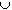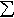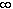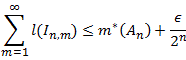#Interactive Real Analysis

Next | Previous | Glossary | Map

## 7.3. Measures

### Proposition 7.3.4: Properties of Outer Measure

Outer measure has the following properties:
1. Outer measure m* is a non-negative set function whose domain is P(R), i.e. the power set of R.
2. The outer measure of an interval is its length.
3. Outer measure is subadditive, i.e. m*(AB)m*(A) + m*(B)
4. Outer measure is countably subadditive, i.e. if { An } is a countable collection of sets, then
m*(An)m*(An)

### Proof

The first statement is obvious. Outer measure is clearly defined for every set, therefore its domain is P(R). It is also non-negative because all terms involved in the inf are non-negative.

We have already shown that the outer measure of open and closed intervals is their length, so we do not have to prove the second statement again.

The third statement is a warmup exercise for the last statement. Because of the definition of outer measure as infimum, we can find open covers {Ai} of A and {Bi} of B, respectively, such thatl(Ai)m*(A) +/2 andl(Bi)m*(B) +/2

But the combined collection {Ai, Bi} covers AB so that

m*(AB)l(Ai) +l(Bi)m*(A) +/2 + m*(B) +/2 = m*(A) + m*(B) +Since this is true for allit proves the third statement.

The proof of the fourth statement, which compared to the previous one might look intimidating because it uses "double indices", is really not that bad: it just switches from adding two terms to adding countably many terms by way of the geometric series. First note that if any of the m*(An) was infinite, the inequality would obviously be true. Thus, we may assume that

m*(An) <for all n

Next, because of the definition of outer measure as an infimum, we know that for any set An, where n is some fixed index, there is a sequence of countably many open intervals In,m such that AnIn,m, where the union is over all m with n fixed, and also, for any> 0:for our fixed n. But now we see that the collection of all (countably many) In,m, ordered somehow, covers A:so that, using the infimum property again:Since this inequality is valid for every> 0, it proves subadditivity.

[ x ]
Next | Previous | Glossary | Map